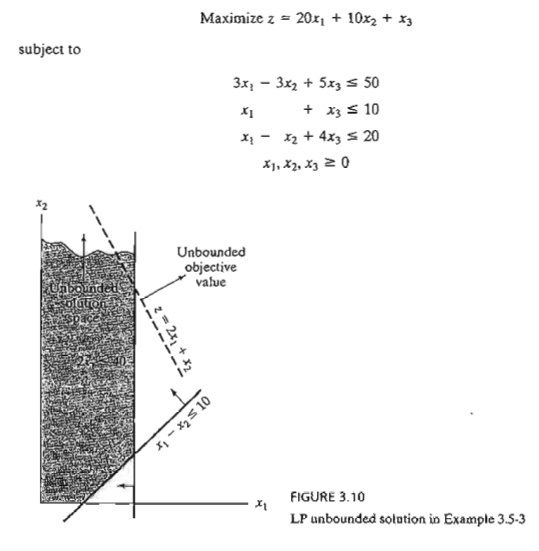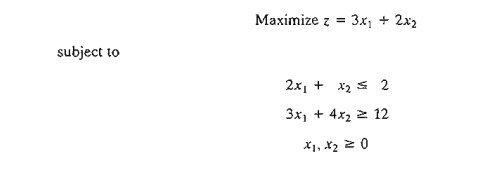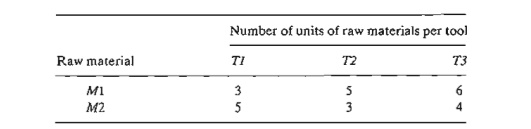Home | | Resource Management Techniques | Special Cases in the Simplex Method

# Special Cases in the Simplex Method

This section considers four special cases that arise in the use of the simplex method. 1. Degeneracy 2. Alternative optima 3. Unbounded solutions 4. Nonexisting (or infeasible) solutions

SPECIAL CASES IN THE SIMPLEX METHOD

This section considers four special cases that arise in the use of the simplex method.

1.     Degeneracy

2.     Alternative optima

3.     Unbounded solutions

4.     Nonexisting (or infeasible) solutions

Our interest in studying these special cases is twofold: (1) to present a theoretical explanation of these situations and (2) to provide a practical interpretation of what these special results could mean in a real-life problem.

1. Degeneracy

In the application of the feasibility condition of the simplex method, a tie for the mini-mum ratio may occur and can be broken arbitrarily. When this happens, at least one basic variable will be zero in the next iteration and the new solution is said to be degenerate.

There is nothing alarming about a degenerate solution, with the exception of a small theoretical inconvenience, called cycling or circling, which we shall discuss short-ly. From the practical standpoint, the condition reveals that the model has at least one redundant constraint. To provide more insight into the practical and theoretical im-pacts of degeneracy, a numeric example is used.

Example 3.5-1       (Degenerate Optimal Solution)Given the slack variables x3 and x4, the following tableaus provide the simplex iterations of the problem:In iteration 0, x3 and x4 tie for the leaving variable, leading to degeneracy in iteration 1 because the basic variable x4 assumes a zero value. The optimum is reached in one additional iteration.

What is the practical implication of degeneracy? Look at the graphical solution in Figure 3.7. Three lines pass through the optimum point (x1 = 0, x2 = 2). Because this is a two-dimensional problem, the point is overdetermined and one of the constraints is redundant? In practice, the mere knowledge that some resources are superfluous can be valuable during the implementa-tion of the solution. The information may also lead to discovering irregularities in the construc-tion of the model. Unfortunately, there are no efficient computational techniques for identifying the redundant constraints directly from the tableau.

From the theoretical standpoint, degeneracy has two implications. The first is the phe-nomenon of cycling or circling. Looking at simplex iterations 1 and 2, you will notice that the objective value does not improve (z = 18). It is thus possible for the simplex method to enter a repetitive sequence of iterations, never improving the objective value and never satisfying the optimality condition (see Problem 4, Set 3.5a). Although there are methods for eliminat-ing cycling, these methods lead to drastic slowdown in computations. For this reason, most LP codes do not include provisions for cycling, relying on the fact that it is a rare occurrence in practice.

The second theoretical point arises in the examination of iterations 1 and 2. Both iterations, though differing in the basic-nonbasic categorization of the variables, yield identical values for all the variables and objective value-namely,

x1=0, x2= 2, x3= 0, x4=0,  z=18

Is it possible then to stop the computations at iteration 1 (when degeneracy first appears), even though it is not optimum? The answer is no, because the solution may be temporarily de-generate as Problem 2, Set 3.5a demonstrates.

PROBLEM SET 3.5A

*1. Consider the graphical solution space in Figure 3.8. Suppose that the simplex iterations start at A and that the optimum solution occurs at D. Further, assume that the objective function is defined such that at A, xI enters the solution first.

a. Identify (on the graph) the corner points that define the simplex method path to the optimum point.

b. Determine the maximum possible number of simplex iterations needed to reach the optimum solution, assuming no cycling.

2. Consider the following LP:a. Show that the associated simplex iterations are temporarily degenerate (you may use TORA for convenience).

b. Verify the result by solving the problem graphically (TORA's Graphic module can be used here).

3. TORA experiment. Consider the LP in Problem 2.

a. Use TORA to generate the simplex iterations. How many iterations are needed to reach the optimum?

b. Interchange constraints (1) and (3) and re-solve the problem with TORA. How many iterations are needed to solve the problem?

c. Explain why the numbers of iterations in (a) and (b) are different.4. TORA Experiment Consider the following LP (authored by E.M. Beale to demonstrate cycling):From TORA's SOLVEIMODIFY menu, select Solve => Algebraic. => Iterations => All-slack. Next, "thumb" through the successive simplex iterations using the command Next iteration (do not use All iterations, because the simplex method will then cycle in-definitely). You will notice that the starting all-slack basic feasible solution at iteration 0 will reappear identically in iteration 6. This example illustrates the occurrence of cycling in the simplex iterations and the possibility that the algorithm may never converge to the optimum solution.

It is interesting that cycling will not occur in this example if all the coefficients in this LP are converted to integer values by using proper multiples (try it!).

2. Alternative Optima

When the objective function is parallel to a nonredundant binding constraint (i.e., a constraint that is satisfied as an equation at the optimal solution), the objective function can assume the same optimal value at more than one solution point, thus giving rise to alternative optima. The next example shows that there is an infinite number of such solutions. It also demonstrates the practical significance of encoun-tering such solutions.

Example 3.5-2    (Infinite Number of Solutions)Figure 3.9 demonstrates how alternative optima can arise in the LP model when the objec-tive function is parallel to a binding constraint. Any point on the line segment Be represents an alternative optimum with the same objective value z == 10.

The iterations of the model are given by the following tableaus.Iteration 1 gives the optimum solution x1 = 0, x2 = 5/2 and z = 10, which coincides with point B in Figure 3.9. How do we know from this tableau that alternative optima exist? Look at the z-equation coefficients of the nonbasic variables in iteration 1. The coefficient of nonbasic x1 is zero, indicating that x1 can enter the basic solution without changing the value of z, but causing a change in the values of the variables. Iteration 2 does just that-letting x1 enter the basic solution and forcing x4 to leave. The new solution point occurs at C(xI = 3, x2 = 1, z = 10). (TORA's Iteratioris option allows determining one alternative optimum at a time.)

The simplex method determines only the two corner points Band C. Mathematically, we can determine all the points (x1, x2) on the line segment Be as a nonnegative. weighted average of points Band C. Thus, givenRemarks. In practice, alternative optima are useful because we can choose from many solutions without experiencing deterioration in the objective value. For instance, in the present ex-ample, the solution at B shows that activity 2 only is at a positive level, whereas at C both activities are positive. If the example represents a product-mix situation, there may be advan-tages in producing two products rather than one to meet market competition. In this ease, the so-lution at C may be more appealing.

PROBLEM SeT 3.5B

*1. For the following LP, identify three alternative optimal basic solutions, and then write a general expression for all the nonbasic alternative optima comprising these three basic solutions.Note: Although the problem has more than three alternative basic solution optima, you are only required to identify three of them. You may use TORA for convenience.

2. Solve the following LP:From the optimal tableau, show that all the alternative optima are not corner points (i.e., nonbasic). Give a two-dimensional graphical demonstration of the type of solu-tion space and objective function that will produce this result. (You may use TORA for convenience.)

3. For the following LP, show that the optimal solution is degenerate and that none of the alternative solutions are corner points (you may use TORA for convenience).3. Unbounded Solution

In some LP models, the values of the variables may be increased indefinitely without violating any of the constraints-meaning that the solution space is unbounded in at least one variable. As a result, the objective value may increase (maximization case) or decrease (minimization case) indefinitely. In this case, both the solution space and the optimum objective value are unbounded.

Unboundedness points to the possibility that the model is poorly constructed. The most likely irregularity in such models is that one or more nonredundant constraints have not been accounted for, and the parameters (constants) of some constraints may not have been estimated correctly.

The following examples show how unboundedness, in both the solution space and the objective value, can be recognized in the simplex tableau.In the starting tableau, both xl and x2 have negative z-equation coefficients. Hence either one can improve the solution. Because xl has the most negative coefficient, it is normally selected as the entering variable. However, all the constraint coefficients under x2 (Le., the denominators of the ratios of the feasibility condition) are negative or zero. This means that there is no leaving variable and that x2 can be increased indefinitely without violating any of the constraints (compare with the graphical interpretation of the minimum ratio in Figure 3.5). Because each unit increase in xl will increase z by 1, an infinite increase in x2 leads to an infinite increase in z.

Thus, the problem has no bounded solution. This result can be seen in Figure 3.10. The solution space is unbounded in the direction of x2, and the value of z can be increased indefinitely. Remarks. What would have happened if we had applied the strict optimality condition that 3.5 calls for xl to enter the solution? The answer is that a succeeding tableau would eventually have led to an entering variable with the same characteristics as x2. See Problem 1, Set3.5c.

PROBLEM SET 3.5C

1. TORA Experiment. Solve Example 3.5-3 using TORA's Iterations option and show that even though the solution starts with xl as the entering variable (per the optimality condition), the simplex algorithm will point eventually to an unbounded solution.

*2. Consider the LP:a. By inspecting the constraints, determine the direction (xI. x2, or x3) in which the solution space is unbounded.

b. Without further computations, what can you conclude regarding the optimum objective value?

3. In some ill-constructed LP models, the solution space may be unbounded even though the problem may have a bounded objective value. Such an occurrence can point only to irregularities in the construction of the model. In large problems, it may be difficult to detect unboundedness by inspection. Devise a procedure for determining whether or not a solution space is unbounded.

4. Infeasible Solution

LP models with inconsistent constraints have no feasible solution. This situation can never occur if all the constraints are of the type with nonnegative right-hand sides because the slacks provide a feasible solution. For other types of constraints, we use artificial variables. Although the artificial variables are penalized in the objective function to force them to zero at the optimum, this can occur only if the model has a feasible space. Otherwise, at least one artificial variable will be positive in the optimum iteration. From the practical standpoint, an infeasible space points to the possibility that the model is not formulated correctly.

Example 3.5-4         (Infeasible Solution Space)

Consider the following LP:Using the penalty M = 100 for the artificial variable R, the following tableaux provide the simplex iterations of the model.Optimum iteration 1 shows that the artificial variable R is positive (= 4), which indicates that the problem is infeasible. Figure 3.11 demonstrates the infeasible solution space. By allowingthe artificial variable to be positive, the simplex method, in essence, has reversed the direction of the inequality from 3x1 + 4x2 ≥ 0: 12 to 3xl + 4x2 12 (can you explain how?). The result is what we may call a pseudo-optimal solution.

PROBLEM SET 3.50

*1. Tooleo produces three types of tools, T1, T2, and T3. The tools use two raw materials, M1 and M2, according to the data in the following table:The available daily quantities of raw materials M1 and M2 are 1000 units and 1200 units, respectively. The marketing department informed the production manager that according to their research, the daily demand for all three tools must be at least 500 units. Will the manufacturing department be able to satisfy the demand? If not, what is the most Toolco can provide of the three tools?

2. TO RA Experiment. Consider the LP modelUse TORA's Iterations => M-Meth6d to show that the optimal solution includes an artificial basic variable, but at zero level. Does the problem have a feasible optimal solution?

Study Material, Lecturing Notes, Assignment, Reference, Wiki description explanation, brief detail
Operations Research: An Introduction : The Simplex Method and Sensitivity Analysis : Special Cases in the Simplex Method |